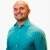# SAT Prep Learn to determine the distance between a point and a lineThis lesson will look at an example problem involving distance. This lesson will give an explanation of how to determine the distance between a point and a line with a step by step look to better... This lesson will look at an example problem involving distance. This lesson will give an explanation of how to determine the distance between a point and a line with a step by step look to better explain the concept.
More... Collapse
9 Views